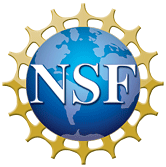XXXV Workshop on Geometric Methods in Physics 26.06-2.07.2016
 Home First Announcement Second Announcement Travel Participants of Workshop Participants of School Registration Photos Proceedings Previous Workshops Email

Anatolij Antonevich

QUASI-PERIODIC ALGEBRAS AND THEIR AUTOMORPHISMS

Let $CB(\mathbb{R}^m)$ be the space of bounded continuous functions on $\mathbb{R}^m$. A closed subalgebra $\mathcal{A}\subset CB(\mathbb{R}^m)$ is called a quasi-periodic algebra, if it is generated by a finite number of exponent
$$e^{i 2 \pi <\pm h_j, x>}, \ h_j \in \mathbb{R}^m, j =1,2, \ldots N.$$ Quasi-periodic algebras and quasi-periodic functions arise in the theory of quasi-crystals and other problems.

 Event sponsored by:Webpage by: Tomasz Golinski